Cantilever Deflection (I need help) - HP50
11-22-2020, 04:14 PM (This post was last modified: 11-22-2020 04:34 PM by CMarangon.)
Post: #1CMarangonMember Posts: 107 Joined: Oct 2020
Cantilever Deflection (I need help) - HP50
Hello!

I can solve this problem, but result seems to be a little large.

PRELIMINARY
Set Mode RPN , flag 117, Checked for SOFT MENU.

Acess Equation Library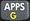So access
after

So will appear a Equation Library menu, so go to:

COLUMNS AND BEAMS
and press ENTER
now choose
CANTILEVER DEFLECTION

Problem 01
Calculate the cantilever deflection
at the edge of a beam of steel, of square section,
as shown in the picture, with lenght of 20m.

Data:
L =20m (length)
E = 200 000 000 kPa
(Elasticity Module or Young's Module, for steel E = 200GPa)

I = b.h3/12 = 200 x 300³ /12 = 450 000 000 mm4 (moment of inertia)

a = 10m (distance from wall to the point of load)
c = 12m (distance to app. moment
M = 5kN.m (app moment)
x = 20m (at the edge, x=lenght)

y =? (variable to calculate)

To me t is y = -195 cm.

Is it right?

Pics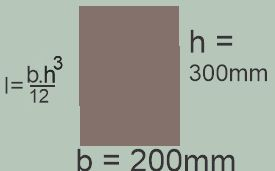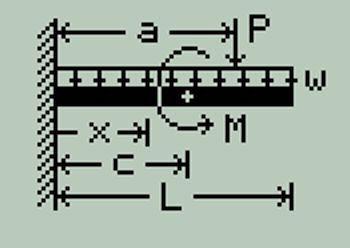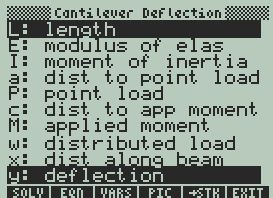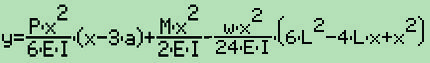Carlos - Brazil
Time Zone: GMT -3
http://area48.com
11-22-2020, 05:59 PM
Post: #2
 GeoffJunior Member Posts: 18 Joined: Apr 2018
RE: Cantilever Deflection (I need help) - HP50
I don't have a 50g, so I can only reply as a civil engineer.

For the values you selected 195 cm is the correct answer. However, the equation you show isn't what's used to arrive at 195 cm.

The first term (involving P) is only correct for x smaller than a. It should be (Pa²/6EI)(3x-a) for x greater than a. If the calculator had used the formula you show it would have reported 192 cm, so I suspect it's using the correct formula somehow.

The second term (involving M) also appears to only be for x smaller than a. A mid-span moment is unusual so I can't easily lay my hands on the correct formula for any x, but I do know the formula for the outer end. This load is only causing about 1 cm of the deflection so any formula error mid-span would be small.

The third term is correct for all x as the load is full length.

In reality a cantilever 20 m long made from such a small beam will fail, and the end deflection will be the distance to wherever it's laying on the ground. < grin >
11-22-2020, 09:51 PM (This post was last modified: 11-22-2020 09:57 PM by CMarangon.)
Post: #3CMarangonMember Posts: 107 Joined: Oct 2020
RE: Cantilever Deflection (I need help) - HP50
(11-22-2020 05:59 PM)Geoff Wrote:  I don't have a 50g, so I can only reply as a civil engineer.

For the values you selected 195 cm is the correct answer. However, the equation you show isn't what's used to arrive at 195 cm.

The first term (involving P) is only correct for x smaller than a. It should be (Pa²/6EI)(3x-a) for x greater than a. If the calculator had used the formula you show it would have reported 192 cm, so I suspect it's using the correct formula somehow.

The second term (involving M) also appears to only be for x smaller than a. A mid-span moment is unusual so I can't easily lay my hands on the correct formula for any x, but I do know the formula for the outer end. This load is only causing about 1 cm of the deflection so any formula error mid-span would be small.

The third term is correct for all x as the load is full length.

In reality a cantilever 20 m long made from such a small beam will fail, and the end deflection will be the distance to wherever it's laying on the ground. < grin >

Hello!

Indeed, I have concluded that these are irreal values for a cantilever.
A cantilever of 20m long and 20cm x 30cm, should not support even its self weight.

I will also calculate its slope. I suppose that it gives a
value able to bent it 90 degrees or more.

At HP-50 AUR, 5-6 (chapter 5, page 6), it gives
a good exmple, but in ENGL units.
All I need to do is convert:-)

Thank you!

Carlos - Brazil
Time Zone: GMT -3
http://area48.com
11-23-2020, 03:02 PM
Post: #4
 GeoffJunior Member Posts: 18 Joined: Apr 2018
RE: Cantilever Deflection (I need help) - HP50
That's funny. I converted your example to Imperial so I could follow it!

These formulas assume fully elastic behavior, meaning they will calculate results (deflection, slope, moment, etc) as though the beam is unbreakable.

I was interested enough to look for the 50g AUR. I see just below the nomenclature for Columns and Beams they note the calculations vary depending on where x is compared to the loads. However in the cantilever deflection formula a couple of pages later only shows one formula. It is correct for the sketch (x less than a and c). The Equation Library must be very clever to fix this on the fly as x varies!

Have fun.
 « Next Oldest | Next Newest »